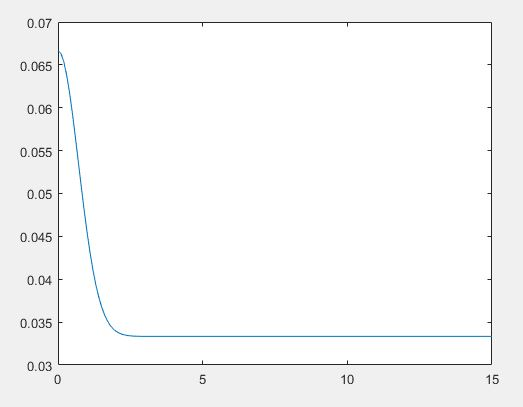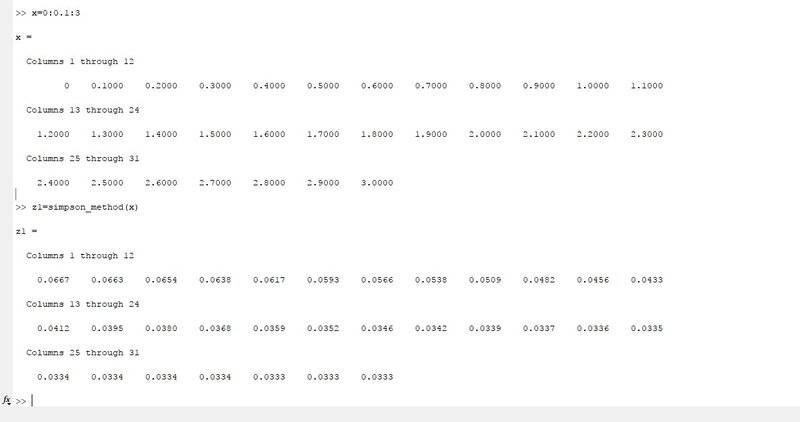# Problem with plotting a function in MATLAB

• diredragon

## Homework Statement

Write code for solving the integral ##\int_{0}^{x}e^{-t^2}dx## using simpsons method and then plot the function from ##x = 0## to ##x = 5## with ##0.1## increment.

## Homework Equations

3. The Attempt at a Solution [/B]
I was told that the best way to plot the function is to write it in Matlab so i decided to give it a go since i haven't really worked in MATLAB before.This is my integral solution code:
Matlab:
function y = simpson_method(x)

y1 = exp(-0.^2);
y2 = exp(-(x).^2);

c = 0;
d = 0;
incr = 0.1;

for i = 1 : (x - 0)/incr - 0.5

y = exp(-(0 + i*incr).^2);

if i/2 == floor(i/2)

d = d + y;
continue
else
c = c + y;
continue
end
end

y = incr/3 * (y1 + 4*c + 2*d + y2);
and the plot code:
Matlab:
x = 0:0.1:15;
y = simpson_method(x);
plot(x,y)
But the output plot is wrong and looks like this:Which is weird since the function i wrote gives correct values at all points. What is wrong here?

#### Attachments

You might want to check whether or not your function gives the correct output when you feed it a vector of several points to evaluate it at.

You might want to check whether or not your function gives the correct output when you feed it a vector of several points to evaluate it at.
I'm not sure how to do that on MATLAB just yet. Is there somewhere i can see examples of that?

That is what you are doing when you first write x = 0:9.1:15 and then function(x). You are passing a vector as argument and your function needs to be able to handle that.

That is what you are doing when you first write x = 0:9.1:15 and then function(x). You are passing a vector as argument and your function needs to be able to handle that.It does output wrong when i input a vector. How do i fix that in the code? How to make a function able to handle a vector?

#### Attachments

You should take a close look at your function. Notice that y1 is always 1. Is that what you want in the last equation for y? I recommend that you simplify the function, add comments to keep it clear, and do not use the variable y inside the loop.

You should take a close look at your function. Notice that y1 is always 1. Is that what you want in the last equation for y? I recommend that you simplify the function, add comments to keep it clear, and do not use the variable y inside the loop.
I made all the corrections and it's still outputting wrong when i feed it a vector like before.
Matlab:
function y = simpson_method(x)  %function which i integrate is e^(-x^2)
yn = exp(-(x).^2);                    %I am using the simpsons method which calculates like this:
%h/3*(f(0) + 4*(f1) + 2*(f(2) + 4*f(3) + ... + f(n))
c = 0;
d = 0;
h= 0.1;

for i = 1 : (x - 0)/h - 0.5                 %summing the parts of the function

f = exp(-(0 + i*h).^2);                %calculating f(i*h)

if i/2 == floor(i/2)                   %checking whether it's even or odd i and adding it to the sum

d = d + f;
continue
else
c = c + f;
continue
end
end

y = h3 * (1+ 4*c + 2*d + yn);         %summing all the parts and return the integral
It has to be that it can't handle a vector but I am not sure how to fix that.

for i = 1 : (x - 0)/h - 0.5 %summing the parts of the function
What do you think this line does?

Edit: I also strongly advice against using i as a variable. It should be reserved for the imaginary unit.

What do you think this line does?
This line is a for loop which increases i from 1 to x/n which is a number of sub-intervals. The -0.5 was added as it does not output correct when i simply write x/n

But the x you input is a vector!

•diredragon
But the x you input is a vector!
Oh i see it now, can't use x. Does it also pose a problem in the definition of yn? Also do i now need somehow to state that only one element at a time from x is used in the loop. How do i do that?

Last edited: